# 12- LINEAR PROGRAMMING

Here we are going through the ncert solutions for class 12 maths chapter 12– linear programming . so before going through the ncert solutions make sure to go through the textbook that helps you to understand the solutions more easily.

# ​​ ​​ ​​ ​​ ​​​​ Linear Programming

#### Question 1:

Maximise Z = 3x + 4y

Subject to the constraints: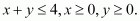The feasible region determined by the constraints, x y ≤ 4, x ≥ 0, y ≥ 0, is as​​ follows.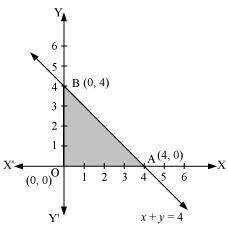The corner points of the feasible region are O (0, 0), A (4, 0), and B (0, 4). The values of Z at these points are as follows.

 Corner point Z = 3x + 4y O(0, 0) 0 A(4, 0) 12 B(0, 4) 16 → Maximum

Therefore, the maximum value of Z is 16 at​​ the point B (0, 4).

Question 2:

Minimise Z = −3x + 4y

subject to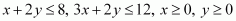.

The feasible region determined by the system of constraints,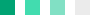x ≥ 0, and y ≥ 0, is as follows.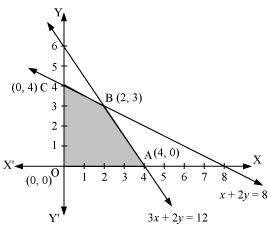The corner points of the feasible region are O (0, 0), A (4, 0), B (2, 3), and C​​ (0, 4).

The values of Z at these corner points are as follows.

 Corner point Z = −3x + 4y 0(0, 0) 0 A(4, 0) −12 → Minimum B(2, 3) 6 C(0, 4) 16

Therefore, the minimum value of Z is −12 at the point (4, 0).

#### Question 3:

Maximise Z = 5x + 3y

subject to.

The feasible region determined by the system of constraints, 3x + 5y ≤ 15,

5x + 2y ≤ 10, x ≥ 0, and y ≥ 0, are as follows.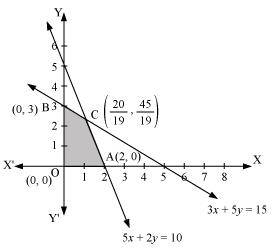The corner points of the feasible region are O (0, 0), A (2, 0), B (0, 3), and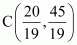.

The values of Z at​​ these corner points are as follows.

 Corner point Z = 5x + 3y 0(0, 0) 0 A(2, 0) 10 B(0, 3) 9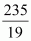→ Maximum

Therefore, the maximum value of Z is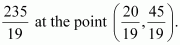#### Question 4:

Minimise Z = 3x + 5y

such that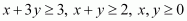.

The feasible region determined by the​​ system of constraints,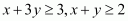, and xy ≥ 0, is as follows.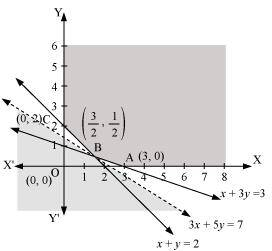It can be seen that the feasible region is unbounded.

The corner points of the feasible region are A (3, 0),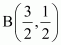, and C (0, 2).

The values of Z at these corner points are as follows.

 Corner point Z = 3x + 5y A(3, 0) 97 → Smallest C(0, 2) 10

As the feasible region is unbounded, therefore, 7 may or may not be the minimum value of Z.

For this, we draw the graph of the inequality, 3x + 5y < 7, and check whether the resulting half plane has​​ points in common with the feasible region or not.

It can be seen that the feasible region has no common point with 3x + 5y < 7 Therefore, the minimum value of Z is 7 at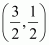.

#### Question 5:

Maximise Z = 3x + 2y

subject to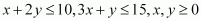.

The feasible region​​ determined by the constraints, x + 2y ≤ 10, 3x + ≤ 15, x ≥ 0, and y ≥ 0, is as follows.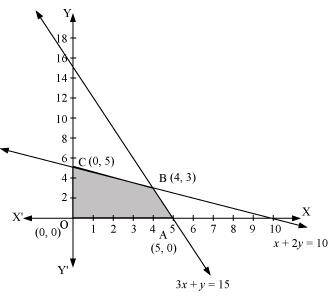The corner points of the feasible region are A (5, 0), B (4, 3), and C (0, 5).

The values of Z at these corner points are as follows.

 Corner point Z = 3x + 2y A(5, 0) 15 B(4, 3) 18 → Maximum C(0, 5) 10

Therefore, the maximum value of Z is 18 at the point (4, 3).

#### Question 6:

Minimise Z = x + 2y

subject to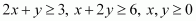.

The feasible region determined by the constraints, 2x + y ≥ 3, x + 2y ≥ 6, x ≥ 0, and y ≥​​ 0, is as follows.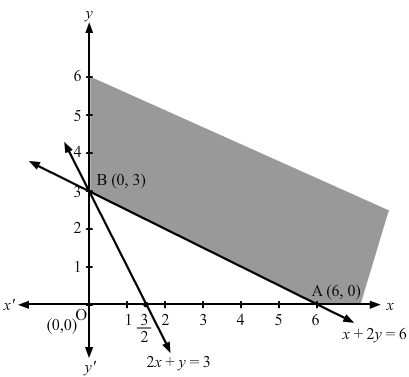The corner points of the feasible region are A (6, 0) and B (0, 3).

The values of Z at these corner points are as follows.

 Corner point Z = x + 2y A(6, 0) 6 B(0, 3) 6

It can be seen that the value of Z at points A and B is same. If​​ we take any other point such as (2, 2) on line x + 2y = 6, then Z = 6

Thus, the minimum value of Z occurs for more than 2 points.

Therefore, the value of Z is minimum at every point on the line, x + 2y = 6

#### Question 7:

Minimise and Maximise Z = 5x + 10y

subject to.

The feasible region determined by the constraints, x + 2y ≤ 120, x + y ≥ 60, x − 2y ≥ 0, x ≥ 0, and y ≥ 0, is as follows.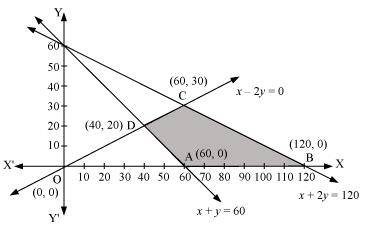The corner points of the feasible region are A (60, 0), B (120, 0), C (60, 30), and D (40, 20).

The​​ values of Z at these corner points are as follows.

 Corner point Z = 5x + 10y A(60, 0) 300 → Minimum B(120, 0) 600 → Maximum C(60, 30) 600 → Maximum D(40, 20) 400

The minimum value of Z is 300 at (60, 0) and the maximum value of Z is 600 at all​​ the points on the line segment joining (120, 0) and (60, 30).

#### Question 8:

Minimise and Maximise Z = x + 2y

subject to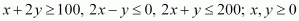.

The feasible region determined by the constraints, x + 2y ≥ 100, 2x − y ≤ 0, 2x + ≤ 200, x ≥ 0, and y ≥ 0, is as​​ follows.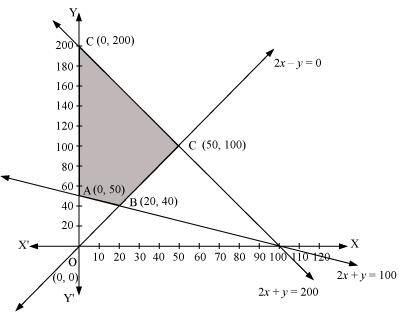The corner points of the feasible region are A(0, 50), B(20, 40), C(50, 100), and D(0, 200).

The values of Z at these corner points are as follows.

 Corner point Z = x + 2y A(0, 50) 100 → Minimum B(20, 40) 100 → Minimum C(50, 100) 250 D(0, 200) 400 → Maximum

The maximum value of Z is 400 at (0, 200) and the minimum value of Z is 100 at all the points on the line segment joining the points (0, 50) and (20, 40).

#### Question 9:

Maximise Z = − x + 2y, subject to the constraints: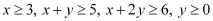.

The feasible region determined by the constraints,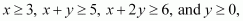is as follows.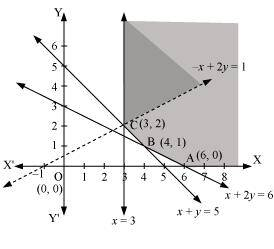It can be seen that the feasible region is unbounded.

The values of Z at corner points A (6, 0), B (4, 1), and C (3, 2) are as follows.

 Corner point Z = −x + 2y A(6, 0) Z = − 6 B(4, 1) Z = − 2 C(3, 2) Z = 1

As the feasible region is unbounded, therefore, Z = 1 may or may not be the maximum value.

For this, we graph the inequality, −x + 2y > 1, and check whether the resulting half plane has points in common with the​​ feasible region or not.

The resulting feasible region has points in common with the feasible region.

Therefore, Z = 1 is not the maximum value. Z has no maximum value.

#### Question 10:

Maximise Z = x + y, subject to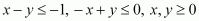.

The region determined by the​​ constraints,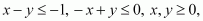is as follows.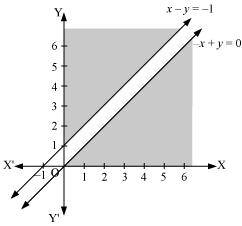There is no feasible region and thus, Z has no maximum value.

#### Question 1:

Reshma wishes to mix two types of food P and Q in such a way that the vitamin contents of the mixture contain at least 8 units of​​ vitamin A and 11 units of vitamin B. Food P costs Rs 60/kg and Food Q costs Rs 80/kg. Food P contains 3 units /kg of vitamin A and 5 units /kg of vitamin B while food Q contains 4 units /kg of vitamin A and 2 units /kg of vitamin B. Determine the minimum cost of the mixture?

Let the mixture contain x kg of food P and y kg of food Q. Therefore,

x ≥ 0 and y ≥ 0

The given information can be compiled in a table as follows.

 Vitamin A (units/kg) Vitamin B (units/kg) Cost (Rs/kg) Food P 3 5 60 Food Q 4 2 80 Requirement (units/kg) 8 11

The mixture must contain at least 8 units of vitamin A and 11 units of vitamin B. Therefore, the constraints are

3x + 4y ≥ 8

5x + 2y ≥ 11

Total cost, Z, of purchasing food is, Z = 60x + 80y

The​​ mathematical formulation of the given problem is

Minimise Z = 60x + 80y … (1)

subject to the constraints,

3x + 4y ≥ 8 … (2)

5+ 2y ≥ 11 … (3)

xy ≥ 0 … (4)

The feasible region determined by the system of constraints is as follows.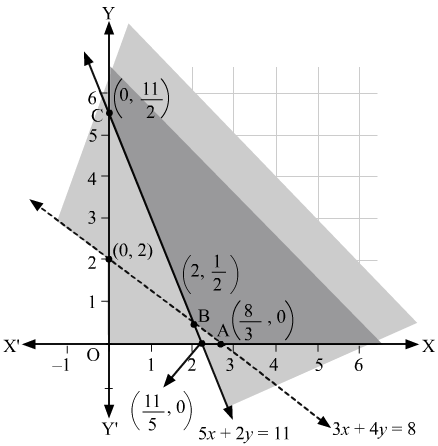It can be seen that the feasible region is unbounded.

The corner points of the feasible region are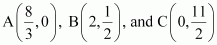.

The values of Z at these corner points are as follows.

 Corner point Z = 60x + 80y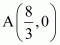160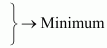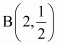160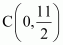440

As the feasible region is unbounded, therefore, 160 may or​​ may not be the minimum value of Z.

For this, we graph the inequality, 60x + 80y < 160 or 3x + 4y < 8, and check whether the resulting half plane has points in common with the feasible region or not.

It can be seen that the feasible region has no​​ common point with 3x + 4y < 8

Therefore, the minimum cost of the mixture will be Rs 160 at the line segment joining the points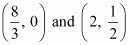.

#### Question 2:

One kind of cake requires 200g flour and 25g of fat, and another kind of cake requires 100g of flour and 50g of fat. Find the maximum number of cakes which can be made from 5 kg of flour and 1 kg of fat assuming that there is no shortage of the other ingredients used in making the cakes?

Let there be x cakes of first kind and y cakes of second kind.​​ Therefore,

x ≥ 0 and y ≥ 0

The given information can be complied in a table as follows.

 Flour (g) Fat (g) Cakes of first kind, x 200 25 Cakes of second kind, y 100 50 Availability 5000 1000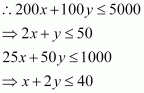Total numbers of cakes, Z, that can be made are, Z = x + y

The mathematical formulation of the given problem is

Maximize Z = x + y … (1)

subject to the constraints,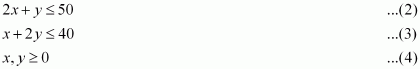The feasible region determined by the system of constraints is as follows.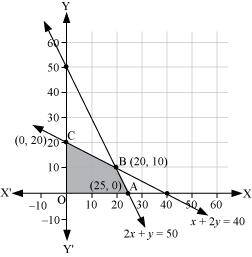The corner points are A (25, 0), B (20, 10), O (0, 0), and C​​ (0, 20).

The values of Z at these corner points are as follows.

 Corner point Z = x + y A(25, 0) 25 B(20, 10) 30 → Maximum C(0, 20) 20 O(0, 0) 0

Thus, the maximum numbers of cakes that can be made are 30 (20 of one kind and 10 of the other​​ kind).

#### Question 3:

A factory makes tennis rackets and cricket bats. A tennis racket takes 1.5 hours of machine time and 3 hours of craftsman’s time in its making while a cricket bat takes 3 hour of machine time and 1 hour of craftsman’s time. In a day, the factory has the availability of not more than 42 hours of machine time and 24 hours of craftsman’s time.

(ii) What number of rackets and bats must be made if the factory is to work at full capacity?

(ii) If the profit on a racket and on a bat is Rs 20 and Rs 10 respectively, find the maximum profit of the factory when it works at full capacity.

(i) Let the number of rackets and the number of bats to be made be x and y respectively.

The machine time is not available for more than 42 hours.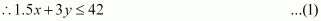The​​ craftsman’s time is not available for more than 24 hours.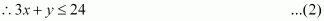The factory is to work at full capacity. Therefore,

1.5x + 3y = 42

3x + y = 24

On solving these equations, we obtain

x = 4 and y = 12

Thus, 4 rackets and 12 bats must be made.

(i) The given​​ information can be complied in a table as follows.

 Tennis Racket Cricket Bat Availability Machine Time (h) 1.5 3 42 Craftsman’s Time (h) 3 1 24

​​ 1.5x + 3y ≤ 42

3x + y ≤ 24

xy ≥ 0

The profit on a racket is Rs 20 and on a bat is Rs 10.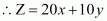The​​ mathematical formulation of the given problem is

Maximize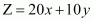… (1)

subject to the constraints,

1.5x + 3y ≤ 42 … (2)

3x + y ≤ 24 … (3)

xy ≥ 0 … (4)

The feasible region determined by the system of constraints is as follows.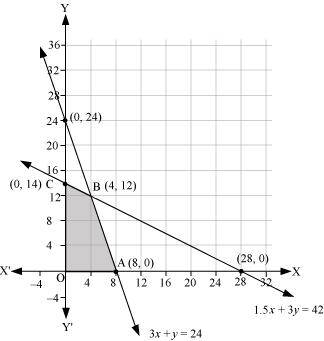The corner points are A (8,​​ 0), B (4, 12), C (0, 14), and O (0, 0).

The values of Z at these corner points are as follows.

 Corner point Z = 20x + 10y A(8, 0) 160 B(4, 12) 200 → Maximum C(0, 14) 140 O(0, 0) 0

Thus, the maximum profit of the factory when it works to its​​ full capacity is Rs 200.

#### Question 4:

A manufacturer produces nuts ad bolts. It takes 1 hour of work on machine A and 3 hours on machine B to produce a package of nuts. It takes 3 hours on machine A and 1 hour on machine B to produce a package of​​ bolts. He earns a profit, of Rs 17.50 per package on nuts and Rs. 7.00 per package on bolts. How many packages of each should be produced each day so as to maximize his profit, if he operates his machines for at the most 12 hours a day?

Let the manufacturer produce x packages of nuts and y packages of bolts. Therefore,

x ≥ 0 and y ≥ 0

The given information can be compiled in a table as follows.

 Nuts Bolts Availability Machine A (h) 1 3 12 Machine B (h) 3 1 12

The profit on a package of nuts is​​ Rs 17.50 and on a package of bolts is Rs 7. Therefore, the constraints are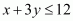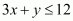Total profit, Z = 17.5x + 7y

The mathematical formulation of the given problem is

Maximise Z = 17.5x + 7y … (1)

subject to the constraints,

x + 3y ≤ 12 … (2)

3x + y ≤ 12 …​​ (3)

xy ≥ 0 … (4)

The feasible region determined by the system of constraints is as follows.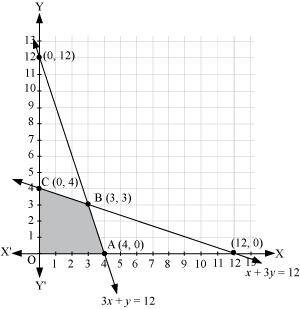The corner points are A (4, 0), B (3, 3), and C (0, 4).

The values of Z at these corner points are as follows.

 Corner point Z = 17.5x + 7y O(0, 0) 0 A(4, 0) 70 B(3, 3) 73.5 → Maximum C(0, 4) 28

The maximum value of Z is Rs 73.50 at (3, 3).

Thus, 3 packages of nuts and 3 packages of bolts should be produced each day to get the maximum profit of Rs 73.50.

#### Question 5:

A factory​​ manufactures two types of screws, A and B. Each type of screw requires the use of two machines, an automatic and a hand operated. It takes 4 minutes on the automatic and 6 minutes on hand operated machines to manufacture a package of screws A, while it takes 6 minutes on automatic and 3 minutes on the hand operated machines to manufacture a package of screws B. Each machine is available for at the most 4 hours on any day. The manufacturer can sell a package of screws A at a profit of Rs 7 and screws B at a​​ profit of Rs10. Assuming that he can sell all the screws he manufactures, how many packages of each type should the factory owner produce in a day in order to maximize his profit? Determine the maximum profit.

Let the factory manufacture x screws of type A and y screws of type B on each day. Therefore,

x ≥ 0 and y ≥ 0

The given information can be compiled in a table as follows.

 Screw A Screw B Availability Automatic Machine (min) 4 6 4 × 60 =240 Hand Operated Machine (min) 6 3 4 × 60 =240

The profit on a package of screws A is Rs 7 and on the package of screws B is Rs 10. Therefore, the constraints are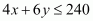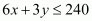Total profit, Z = 7x + 10y

The mathematical formulation of the given problem is

Maximize Z = 7x + 10y … (1)

subject to the​​ constraints,… (2)… (3)

xy ≥ 0 … (4)

The feasible region determined by the system of constraints is